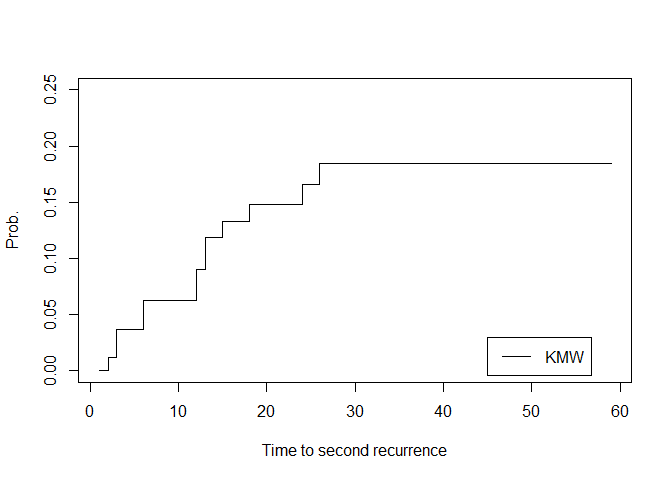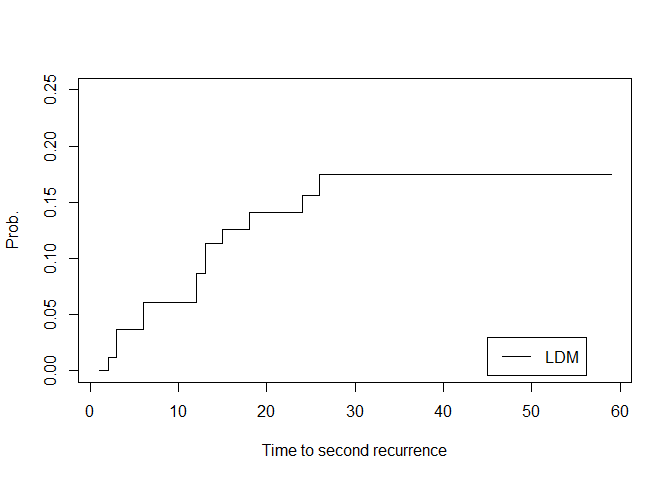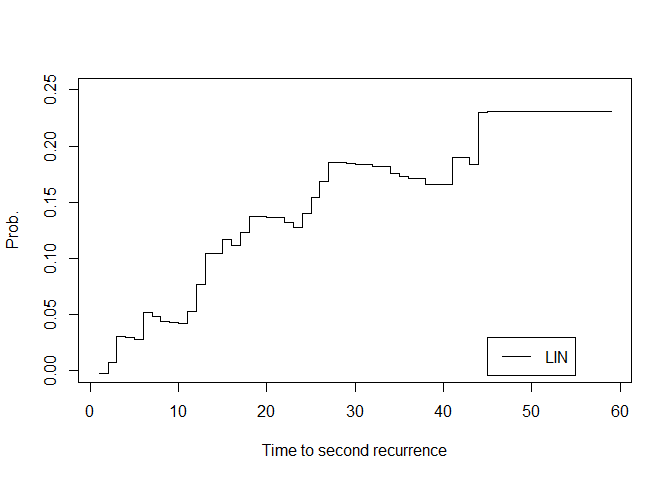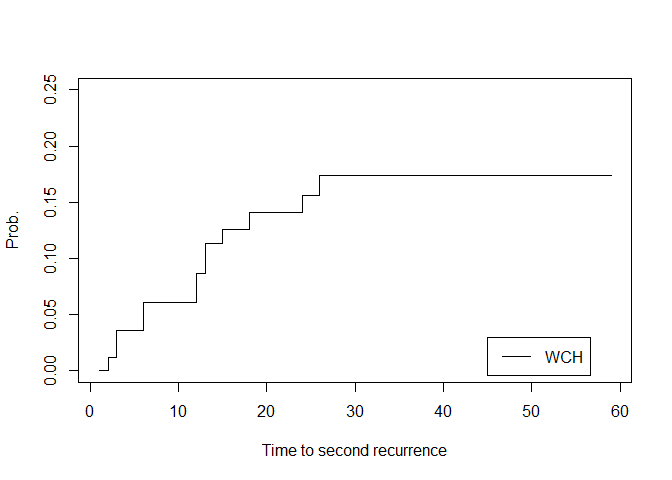# survivalREC: Nonparametric Estimation of the Distribution of Gap Times for Recurrent Events

#### 2022-03-14

The purpose of the present vignette is to demonstrate the capacities of the R package survivalREC, as a tool to get nonparametric estimation of the distribution of gap times for recurrent events. The methods implemented in the package are applied to the study of (multiple) recurrence times in patients with bladder tumors.

## Recurrent events

In many longitudinal studies, subjects can experience recurrent events (Cook 2007). This type of data has been frequently observed in medical research, engineering, economy and sociology. In medical research, the recurrent events could be multiple occurrences of hospitalization from a group of patients, multiple recurrence episodes in cancer studies, recurrent upper respiratory and ear infections, repeated heart attacks or multiple relapses from remission for leukemia patients. The analysis of such data can be focused on time-between-events (gap times) or time-to-event models. In time-to-event models, the events of concern usually represent different states in the disease process (e.g. alive and disease-free, alive with disease and dead) and they are modeled through their intensity functions (Andersen et al 1993, Meira- Machado et al 2009, Meira-Machado and Sestelo, 2019). In this vignette we consider that the events are of the same nature and focus on time-between-events.

## Estimators

Four different approaches for estimating the bivariate distribution function of $$(Y_1,Y_2)$$, $$F_{12}(y_1,y_2)=P(Y_1\leq y_1,Y_2\leq y_2)$$ are implemented in the survivalREC package. The generalization of these methods to more than two gap times is also considered.

The package implements the bivariate estimators proposed in Lin, Sun and Ying (1999) and de Uña-Álvarez and Meira-Machado (2008). In both estimators right censoring is handled by appropriate reweighting of the chosen summands and the differences between the two estimators are somewhat subtle in this regard. The second estimator (labeled as KMW) only put mass on observations that are completely uncensored (i.e., fully observed till the second event) whereas the first estimator (labeled as LIN) jumps on observations that were uncensored till a given time. In practice this means that Lin’s estimator will show estimated curves with more jump points. The estimates produced via the second estimator produce a valid bivariate distribution since it does guarantee that the bivariate distribution function is monotone. In contrast, the specific reweighting of the data which is used in Lin’s estimator do not ensure this property. Their estimators do not attach positive mass to each pair of recorded gap times, which may lead to problems of interpretation.

A simple estimator for the bivariate distribution function of $$(Y_{1},Y_2)$$ based on subsampling. The estimator (labeled as LDM) considers the relation, $$P(Y_{1}\leq y_1, Y_{2}\leq y_2) = P(Y_{2}\leq y_2\vert Y_{1}\leq y_1)P(Y_{1}\leq y_1)$$, where the second term in the right-hand side of the equation can be estimated using the Kaplan-Meier product-limit estimator of the distribution function of the first event time. The first term can be estimated using a subsampling approach.

Any of the previous estimators proposed above (LIN, KMW and LDM) may reveal some problems in the right tail where uncensored observations are scarce. Below we propose an estimator that may deal more efficiently at those situations. The proposed estimator is constructed using the cumulative hazard of the total time given a first time but where each observation has been weighted using the information of the first duration. This estimator (WCH - weighted cumulative hazard) follow the ideas by Wang and Wells (1998) in which a product-limit estimator for the second gap time is used.

## Application to Bladder Cancer Study Data

We will use data on the 85 subjects with nonzero follow-up who were assigned to either thiotepa or placebo, with respective sizes of 47 and 38. Among the 85 patients, 47 relapsed at least once, among these, 29 had a second recurrence, 22 had a third recurrence and 14 had four or more recurrences (16.5%). Thus, in our study only the first three recurrence times $$T_1$$, $$T_2$$ and $$T_3$$ (or the corresponding gap times $$Y_1$$, $$Y_2$$ and $$Y_3$$) are considered. Data sets considering two, three and four recurrences are available in the survivalREC package. To illustrate our methods we will use data with only the first three recurrences for any patient. Bellow, is an excerpt of the data.frame with one row per individual.

library(survivalREC)
#>   id y1 d1 y2 d2 y3 d3 rx size
#> 1  1  1  0  0  0  0  0  1    3
#> 2  2  4  0  0  0  0  0  1    1
#> 3  3  7  0  0  0  0  0  1    1
#> 4  4 10  0  0  0  0  0  1    1
#> 5  5  6  1  4  0  0  0  1    1
#> 6  6 14  0  0  0  0  0  1    1
#>  85  9

The movement among the recurrent events is given by the variables $$y_i$$ and $$d_i$$, with $$i=\lbrace1,\cdots, 4\rbrace$$, which represent, respectively, the four gap times and their corresponding censoring indicators (1 for an event and 0 for censoring). The other three variables are the patient id (id), the type of treatment (rx, 1 = placebo and 2 = thiotepa), and the size (cm) of the largest initial tumour (size).

In what follows, we introduce some examples of how to implement the estimation of the bivariate and distribution functions with three gap times using the survivalREC package. First, we need to transform the original data set into a ´multidf´ format class. This can be done using the multidf function that has as arguments time1, time, event1 and status. These arguments correspond to the soujorn time in the initial state and the global time, as well as the censoring indicators variables for the first transition and the ultimate state. In the following input codes, covariate size was also included.

b3state<-multidf(gap1=bladder4state$y1, event1=bladder4state$d1,
gap2=bladder4state$y2,status=bladder4state$d2,
size=bladder4state$size) class(b3state) #>  "multidf" b3state[][1:10,] #> time1 time event1 status size #> 1 1 1 0 0 3 #> 2 4 4 0 0 1 #> 3 7 7 0 0 1 #> 4 10 10 0 0 1 #> 5 6 10 1 0 1 #> 6 14 14 0 0 1 #> 7 18 18 0 0 1 #> 8 5 18 1 0 3 #> 9 12 16 1 1 1 #> 10 23 23 0 0 3 To obtain the nonparamentric estimates for bivariate distribution, we have the KMWdf, LDMdf, LINdf and WCHdf functions. As an example, suppose we are interested in obtaining the estimates for two gap times, $$x=13$$ and $$y=20$$ for methods KMW, LDM, LIN and WCH. The input codes for this case are the following KMWdf(b3state,x=13,y=20) #>  0.2878889 LDMdf(b3state,x=13,y=20) #>  0.2831524 LINdf(b3state,x=13,y=20) #>  0.2881169 WCHdf(b3state,x=13,y=20) #>  0.2873464 It is also possible to show the estimated curves of the bivariate distribution function given a specific value for the first gap time. This can be done through the plot function for multidf objects. plot(x=b3state, t1=3, method="KMW", type = "s", ylab='Prob.', ylim=c(0,.25), xlab='Time to second recurrence') legend(45,0.03 , legend=c("KMW"), col=c("Black"), lty=1, cex=1)plot(x=b3state, t1=3, method="LANDMARK", type = "s", ylab='Prob.', ylim=c(0,.25), xlab='Time to second recurrence') legend(45,0.03 , legend=c("LDM"), col=c("Black"), lty=1, cex=1)plot(x=b3state, t1=3, method="LIN", type = "s", ylab='Prob.', ylim=c(0,.25), xlab='Time to second recurrence') legend(45,0.03 , legend=c("LIN"), col=c("Black"), lty=1, cex=1)plot(x=b3state, t1=3, method="WCH", type = "s", ylab='Prob.', ylim=c(0,.25), xlab='Time to second recurrence') legend(45,0.03 , legend=c("WCH"), col=c("Black"), lty=1, cex=1)### Nonparametric estimation of the conditional bivariate distribution function The survivalREC package also allows for the computation of nonparametric estimators for bivariate distribution functions conditional on a given continuous covariate. As an example, let’s consider we are interested in computing the estimates for the bivariate distribution with the gap times $$x=13$$ and $$y=15$$ conditional on a tumour size of 3 cm. In the following input code, we also compare the estimates, taking into account the type of the kernel density bandwidth (using the dpik function of the KernSmooth package (by default) or through a specific value bw=2). Both of them have a gaussian density kernel. library(KernSmooth) #> KernSmooth 2.23 loaded #> Copyright M. P. Wand 1997-2009 IPCWdf(object=b3state, x=13, y=15, covariate="size", cov.value=3, window = "gaussian") #>  0.6665688 IPCWdf(object=b3state, x=13, y=15, covariate="size", bw=2, cov.value=3, window = "gaussian") #>  0.4324027 ### Extension to more than two gap times The procedures to extend the estimation to three gap times distribution functions, for the methods KMW, LDM, LIN and WCH, are quite similar to the bivariate case. Primary, we must create a new object using the multidf function, called for this example b4state. As we can see, the b4state object give us the cumulative gap times time1, time2 and time, as well as, the corresponding censoring indicators (event1, event2 and status). Finally, to obtain the estimates, we use the KMW3df, LDM3df, LIN3df and WCH3df functions by including a new parameter $$z$$ to the third gap time. b4state<-multidf(gap1=bladder4state$y1, event1=bladder4state$d1, gap2=bladder4state$y2, event2=bladder4state$d2, gap3=bladder4state$y3, status=bladder4state$d3, size=bladder4state$size)

b4state[][1:10,]
#>    time1 time2 time event1 event2 status size
#> 1      1     1    1      0      0      0    3
#> 2      4     4    4      0      0      0    1
#> 3      7     7    7      0      0      0    1
#> 4     10    10   10      0      0      0    1
#> 5      6    10   10      1      0      0    1
#> 6     14    14   14      0      0      0    1
#> 7     18    18   18      0      0      0    1
#> 8      5    18   18      1      0      0    3
#> 9     12    16   18      1      1      0    1
#> 10    23    23   23      0      0      0    3

KMW3df(b4state,x=13,y=15,z=10)
#>  0.286572

LDM3df(b4state,x=13,y=15,z=10)
#>  0.2144355

LIN3df(b4state,x=13,y=15,z=10)
#>  0.2238439

WCH3df(b4state,x=13,y=15,z=10)
#>  0.2218412